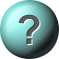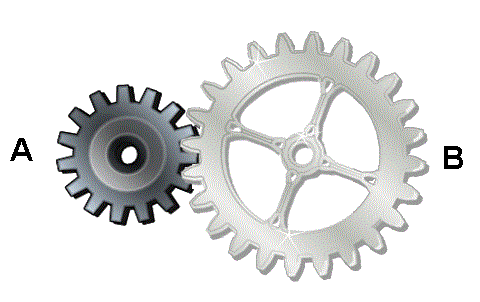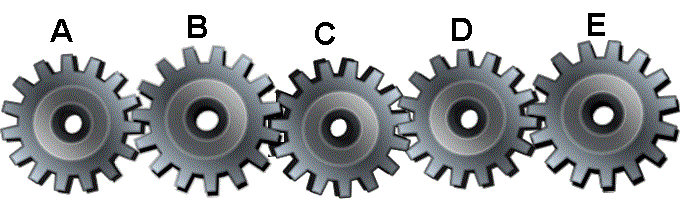### Mechanical Aptitude 6 - Gears More Tests for pullies, levers, circuits and water will be uploaded soon1.    If drive wheel A rotates clockwise at a speed of 10 rpm, how does wheel B turnclockwise slower than Aclockwise faster than Aanti clockwise slower than AAnti clockwise faster than A2.    Last gear will move clockwise if?Gear D is moving in clockwise direction.Gear B & C are moving in clockwise direction.Gear A is moving in clockwise direction.Gear B is moving in clockwise direction.3.    Gear D will move in anti clockwise direction if?Gear C is moving in anti clockwise direction.Gear B is moving in clockwise direction.Gear E is moving in anti clockwise direction.Gear A is moving in iclockwise direction.4.    If gears in the picture are moving then?Both gears will be moving at the same speed.Smaller gear will be moving faster.Gear A will be moving slower.Gear B will be moving faster.5.    Gear B turns clockwise at a constant speed of 30 rpm, how does gear A turn, if the number of teeth in gear A are 8 and in gear B are 24?Anti lockwise 90 rpmClockwise 30 rpmAnti lockwise 60 rpmClockwise 90 rpm

This is more feedback!
This is the feedback!